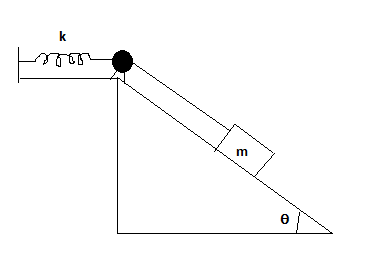# A m = 2.0 kg breadbox on a frictionless incline of angle \theta = 40^\circ is connected, by a...

## Question:

A m = 2.0 kg breadbox on a frictionless incline of angle {eq}\theta = 40^\circ {/eq} is connected, by a cord that runs over a pulley, to a light spring of spring constant k = 120 N/m, as shown in the figure below. The box is released from rest when the spring is not stretched Assume that the pulley is massless and frictionless.

Determine:

a. the speed of the box when it has moved 10 cm down the incline.

b. How far down the incline from its point of release the box slides before momentarily stopping?

c. The magnitude and direction (up or down the incline) of the box's acceleration at the instant the box stops momentarily.## Hooke's Law:

Hooke's Law is a concept on physics discuss the force that compress and expand a spring. From here on we can elaborate a relationship between the force applied and the elasticity of an object. In equation form Hooke's law is,

$$F = - kx$$

where {eq}k {/eq} is the spring constant and {eq}x {/eq} is the length of extension of a spring.

## Answer and Explanation: 1

Become a Study.com member to unlock this answer! Create your account

The list of given we have:

• {eq}m = 2.0\ Kg {/eq}
• {eq}\theta = 40^\circ {/eq}
• {eq}k = 120\ N/m {/eq}

(a) We are ask to find the speed of the...

See full answer below.

#### Learn more about this topic:Hooke's Law & the Spring Constant: Definition & Equation

from

Chapter 4 / Lesson 19
202K

After watching this video, you will be able to explain what Hooke's Law is and use the equation for Hooke's Law to solve problems. A short quiz will follow.